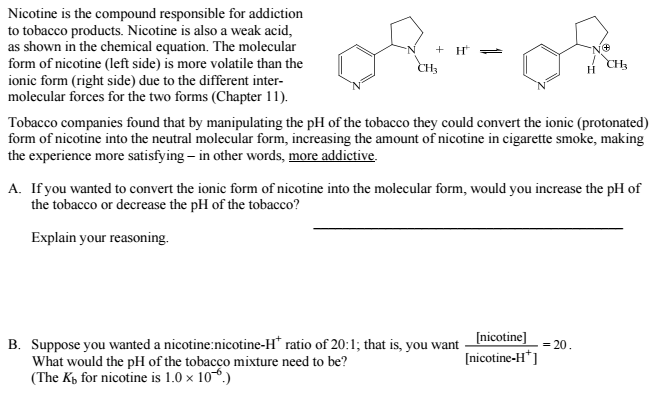# Problem: The Henderson–Hasselbalch (H-H) equation is a clever rearrangement of the  Ka equilibrium expression that comes in very handy for certain pH calculations. The H-H equation can be used to calculate the buffer ratio ([base]/[acid]) required to prepare a buffer at a specific pH. It is possible that the buffer ratio required to prepare a pH 6.85 buffer could be used to study novel chemotherapy agents; however, the noble H-H equation can be used for more nefarious purposes.

###### FREE Expert Solution
100% (200 ratings)
###### Problem Details

The Henderson–Hasselbalch (H-H) equation is a clever rearrangement of the  Ka equilibrium expression that comes in very handy for certain pH calculations. The H-H equation can be used to calculate the buffer ratio ([base]/[acid]) required to prepare a buffer at a specific pH. It is possible that the buffer ratio required to prepare a pH 6.85 buffer could be used to study novel chemotherapy agents; however, the noble H-H equation can be used for more nefarious purposes.Frequently Asked Questions

What scientific concept do you need to know in order to solve this problem?

Our tutors have indicated that to solve this problem you will need to apply the Buffer concept. You can view video lessons to learn Buffer. Or if you need more Buffer practice, you can also practice Buffer practice problems.

What is the difficulty of this problem?

Our tutors rated the difficulty ofThe Henderson–Hasselbalch (H-H) equation is a clever rearran...as high difficulty.

How long does this problem take to solve?

Our expert Chemistry tutor, Jules took 4 minutes and 39 seconds to solve this problem. You can follow their steps in the video explanation above.

What professor is this problem relevant for?

Based on our data, we think this problem is relevant for Professor Dixon's class at UCF.